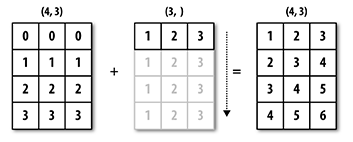# Vectorized Operations

Last updated: March 28th, 2019# Vectorized Operations with NumPy arrays¶

"Vectorization" is an important concept in numeric computing. It refers to the process of operating in entire arrays, in a concise and efficient way. Let's take a look with a couple of examples.

Numpy also refers to vectorized operations as "array broadcasting". We'll use both terms interchangeably.## Hands on!¶

We've seen already some "linear algebra" examples in our previous lessons. These are examples of vectorized operations. For example, given these two arrays:

In [ ]:
import numpy as np

In [ ]:
a = np.arange(0, 5)
a

In [ ]:
b = np.arange(5, 10)
b


A vectorized operation is any algebraic binary operation you perform on them:

In [ ]:
a + b


In this case, the operation was performed "element-wise", ie: "vectorized". Let's compare it with a regular for-loop operation:

In [ ]:
res = []
for i in range(len(a)):
res.append(a[i] + b[i])
np.array(res)


It's much easier to express the operation as a + b, instead of explicitly defining the for loop to perform the element-wise sum. This is the difference between declarative and imperative programming.

Finally, compare this to the usage of regular Python lists:

In [ ]:
l1 = list(range(5))
l1

In [ ]:
l2 = list(range(5, 10))
l2

In [ ]:
l1 + l2


Python lists don't operate "algebraically", the + operation is just concatenation.

Generally speaking, array vectorization will always work with arrays of the same size and shape, but it can also work for arrays of different shapes. For example:

In [ ]:
A = np.array([
[0, 0, 0],
[1, 1, 1],
[2, 2, 2],
[3, 3, 3]
])
A

In [ ]:
A.shape

In [ ]:
b = np.array([[1, 2, 3]])
b

In [ ]:
b.shape


Even though the shapes are different, we can still perform a vectorized operation between A and b:

In [ ]:
A + b


This is possible thanks to the following rule:

The Broadcasting Rule: Two arrays are compatible for broadcasting if for each trailing dimension (i.e., starting from the end) the axis lengths match or if either of the lengths is 1. Broadcasting is then performed over the missing or length 1 dimensions.

Graphically speaking, this is the operation we've performed:Any operation between arrays not following The Broadcasting Rule, will fail with a "broadcast" related error. For example:

In [ ]:
A = np.arange(6).reshape(2, 3)
A

In [ ]:
b = np.array([5, 5])
b

In [ ]:
A + b### Broadcasting with Scalars¶

Vectorized operations between arrays are usually reserved for more "advanced" or "scientific" usages. In your day to day work as a data analyst, you'll be using more often broadcasting with scalars.

Broadcasting with scalars is probably the most intuitive of the vectorized operations:

In [ ]:
a = np.arange(0, 6)
a

In [ ]:
a + 10


As you can see, the + 10 operation was "broadcasted" (or "distributed") among all the elements of the array. This is what we usually do with a list comprehension in pure Python:

In [ ]:
[x + 10 for x in a]


But this "broadcasting" behavior is default for Numpy. Here are a few other examples:

In [ ]:
a - 5

In [ ]:
a * 3


For example, there's a pretty common technique in Machine Learning that's called Standardization, that follows this formula:

$$\large x'={\frac {x-{\bar {x}}}{\sigma }}$$

The objective is to make the data have mean 0, and standard deviation 1. Standarizing an array using Numpy broadcasting is as simple as:

In [ ]:
(a - a.mean()) / a.std()### Vectorized boolean operations¶

As you might know already, aside from regular algebraic operators (+, -), we also have boolean operators. Boolean operations are also broadcasted with arrays. Example:

In [ ]:
a

In [ ]:
a > 2

In [ ]:
a == 0


As you can see, the result of a boolean broadcasted operation, is a boolean array; an array containing the result of applying the boolean operation to each individual element in the array.

A pretty common pattern is to use the function np.sum to count how many elements in the array satisfy a given condition. For example, from our array a, how many elements are greater than 2 (> 2)? The answer is 3 (elements 3, 4 and 5).

In [ ]:
a > 2

In [ ]:
np.sum(a > 2)


Boolean arrays will be very important for masks and filters later, so keep an eye on them.

Finally, it's important to note that vectorized operations (algebraic and boolean) with scalars will also work for multidimensional arrays:

In [ ]:
A = np.arange(1, 10).reshape(3, 3)
A

In [ ]:
A + 100

In [ ]:
A % 2

In [ ]:
A % 2 == 0